<input id="0qass"><u id="0qass"></u></input>
• <input id="0qass"><u id="0qass"></u></input>

# 一、冒泡排序

### 原理與圖示

• 這是一種簡單的排序方法，它使用一種“冒泡策略”把最大元素移到序列最右端
• 下面給出了一次冒泡的過程：在一次冒泡過程中，每相鄰的元素比較，如果左邊的元素大于右邊的元素，則交換。每比較一輪，找出一位最大數，然后移動到序列的最右端。例如下圖是一次冒泡排序的過程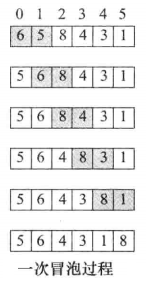• 下面給出了一個總的冒泡過程：每一行表示一次冒泡過程（省略了交換的細節）：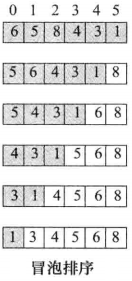### 實現代碼

• 實現代碼如下：
/**
* @description: 冒泡排序, 排序規則(從小到大)
* @param :
arr: 排序的數組
arrSize: 數組的大小
* @return:
* @author: Dongshao
*/
template<typename T>
void bubbleSort(T arr[], size_t arrSize)
{
size_t i, j;

// 外層for循環是冒泡排序執行的排序次數
for (i = 0; i < arrSize - 1; ++i)
{
// 內層for循環是實際冒泡排序的過程
for (j = 0; j < arrSize - 1 - i; ++j)
{
if (arr[j] > arr[j + 1])
{
T temp = arr[j];
arr[j] = arr[j + 1];
arr[j + 1] = temp;

//這三個步驟也可以使用swap(arr[j],arr[j+1]);代替
}
}
}
}

### 測試代碼如下

int main()
{
int arr[] = {5, 4, 1, 2, 7, 4};

bubbleSort<int>(arr, sizeof(arr) / sizeof(int));

for (const auto& elem : arr)
std::cout << elem << " ";

std::cout << std::endl;
}

?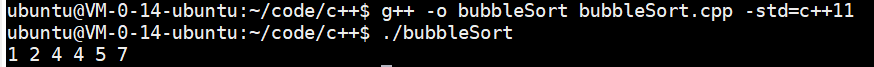# 二、插入排序

### 原理與圖示

• 原理：?首先認為首元素有序，然后從剩余的數據中取出第一個元素與前面的序列進行比較排序，放入到序列中，然后繼續取下一個元素...以此類推
• 例如，下面用白色部分表示有序段，陰影部分表示無序段。插入排序的規則是：
• 第1行排序時，6放在最前面
• 第2行排序時，將無序段的第一個元素5插入到前面白色的有序段中，結果如第2行所示
• 第3行排序時，將無序段的第一個元素8插入到前面白色的有序段中，結果如第3行所示
• ......以此類推，每次從無序段陰影部分挑選出第一個元素，然后插入到前面的白色部分有序段中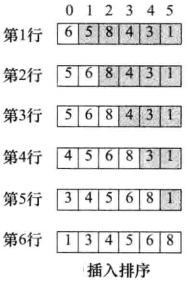• 備注：當然，你可以認為后面有序，前面無序，而每次從前面無序段中插入元素到后面得以有序段中，自己實現，原理是相同的

### 實現方式①

/**
* @description: 插入排序, 排序規則(從小到大)
* @param :
arr: 排序的數組
arrSize: 數組的大小
* @return:
* @author: Dongshao
*/
template<typename T>
void insertionSort1(T arr[], int arrSize)
{
int i, j;

// 從第二個元素開始
for(i = 1; i< arrSize ; i++)
{
// 先記錄下這個數字, 我們要把這個數字插入到前面的序列中
T temp = arr[i];

// 遍歷前面的序列, 找到temp合適插入的位置
// 備注: for循環不能用arr[j] > arr[i]判斷, 因為arr[i]可能會因為排序而改變值
for(j = i- 1; j >= 0 && arr[j] > temp; --j)
arr[j + 1] = arr[j]; // 將大于temp的元素向后移動

// 將這個元素插入
arr[j + 1] = temp;
}
}

### 實現方式②

• 原理與上面是一樣的，只是把內層for循環抽象出來放置到了一個單獨的insert函數中
/**
* @description: 將elem插入到a所在的數組中
* @param :
arr: 排序的數組
index: 指定數組的最終索引
elem: 要插入的元素
* @return:
* @author: Dongshao
*/
template<typename T>
void insert(T arr[], int index, const T& elem)
{
int i;

// 注意, 雖然我們傳入的是index, 但是是從index -1開始向前遍歷的
for (i = index - 1; i >= 0 && arr[i] > elem; --i)
arr[i + 1] = arr[i];

arr[i + 1] = elem;
}

/**
* @description: 插入排序
* @param :
arr: 排序的數組
arrSize: 數組的大小
elem: 要插入的元素
* @return:
* @author: Dongshao
*/
template<typename T>
void insertionSort2(T arr[], int arrSize)
{
int i;
for (i = 1; i < arrSize; ++i)
{
T elem = arr[i];

// 將elem元素插入到arr數組中
insert(arr, i, elem);
}
}

### 測試代碼如下

int main()
{
int arr1[] = {5, 4, 1, 2, 7, 4};
insertionSort1<int>(arr1, sizeof(arr1) / sizeof(int));
std::cout << "arr1: ";
for (const auto& elem : arr1)
std::cout << elem << " ";
std::cout << std::endl;

int arr2[] = {5, 4, 1, 2, 7, 4};
std::cout << "arr2: ";
insertionSort2<int>(arr2, sizeof(arr2) / sizeof(int));
for (const auto& elem : arr2)
std::cout << elem << " ";
std::cout << std::endl;

return 0;
}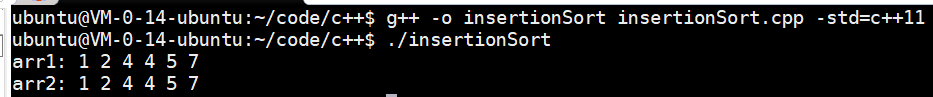# 三、選擇排序

### 原理與圖示

• 原理：對于給定的一個數組，起始從數組中找出一個最大元素放在a[n-1]處，然后再從剩下的n-1個元素中找出最大的元素放在a[n-1]處......以此類推，每次都從前面的剩余序列中找到(選擇)一個最大元素放在后面
• 例如：
• 下面第一行是起始狀態
• 第2行執行時，從所有元素中找出最大的元素8，然后放到最后面
• 第3行執行時，從前面剩余的所有元素中找出最大元素6，然后放到最后面（但是在8前面）
• ......以此類推，完成排序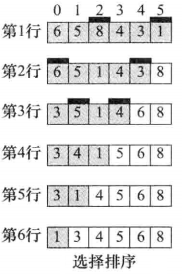• 備注：此處介紹的原理是每次選擇最大的元素然后放到后面，你也可以使用別的方法，例如每次從序列中選擇最小的元素放到前面（原理都是類似的）
• 因為選擇排序每次就是為了從前面的剩余序列中找出最大的元素，因此選擇排序的方式可能有不同種方式，下面給出了兩種實現方法它們的差異在于每次找到最大的元素時是否立即交換

### 實現方式①

• 此種實現方式為：
• 遍歷數組前面的所有元素，用一個max索引記住這個值的位置
• 遍歷完成之后再將這個值放到后面
/**
* @description: 選擇排序, 排序規則(從小到大)
* @param :
arr: 排序的數組
arrSize: 數組的大小
* @return:
* @author: Dongshao
*/
template<typename T>
void selectionSort1(T arr[], int arrSize)
{
int i;
int j;
int max;

// 從最后一個元素開始
for(i = arrSize - 1; i >= 1; i--)
{
// 最大值索引, 先設置為i
max = i;

// 從前面所有的元素中進行查找
for(j = i - 1; j >= 0; j--)
{
// 如果某個值大于arr[max], 就將max索引設置為其索引
if(arr[j] > arr[max])
max = j;
}

// 如果max不與i相等, 說明前面有值比自己大, 那么就交換
if(max != i)
{
T temp = arr[i];
arr[i] = arr[max];
arr[max] = temp;
}
}
}

### 實現方式②

• 此種實現方式為：
• 遍歷數組前面的所有元素，每遍歷到一個大于后面的元素就立即進行交換
• 因為這種實現方法復雜度比實現方法①的復雜度高
/**
* @description: 選擇排序, 排序規則(從小到大)
* @param :
arr: 排序的數組
arrSize: 數組的大小
* @return:
* @author: Dongshao
*/
template<typename T>
void selectionSort2(T arr[], int arrSize)
{
int i;
int j;

// 從最后一個元素開始
for(i = arrSize - 1; i > 1; i--)
{
// 從前面的元素中查找, 只要找到一個比temp大的, 就立即交換
for(j = i - 1; j >= 0; j--)
{
if(arr[j] > arr[i])
{
T temp = arr[i];
arr[i] = arr[j];
arr[j] = temp;
}
}
}
}

### 測試代碼如下

int main()
{
int arr1[] = {5, 4, 1, 2, 7, 4};
selectionSort1<int>(arr1, sizeof(arr1) / sizeof(int));
std::cout << "arr1: ";
for (const auto& elem : arr1)
std::cout << elem << " ";
std::cout << std::endl;

int arr2[] = {5, 4, 1, 2, 7, 4};
selectionSort2<int>(arr2, sizeof(arr2) / sizeof(int));
std::cout << "arr2: ";
for (const auto& elem : arr2)
std::cout << elem << " ";
std::cout << std::endl;

return 0;
}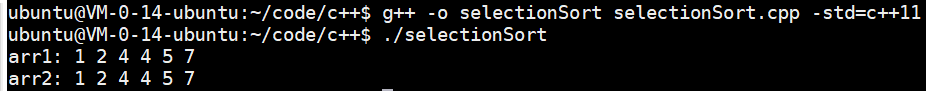# 四、希爾排序

### 原理與圖示

• 希爾排序介紹：希爾（Shell）排序是D.L.shell于1959年提出的，它屬于插入排序方法，是不穩定的排序方法
• 大致原理：希爾排序是把記錄按下標的一定增量分組，對每組使用直接插入排序算法排序；隨著增量逐漸減少，每組包含的關鍵詞越來越多，當增量減至1時，整個文件恰被分成一組，算法便終止
• 排序步驟，以下圖為例：
• 第一次排序時，元素個數總共為12個，那么先設置增量為12/2=6，此時每間隔距離為6的一組元素進行插入排序，例如a與a進行比較、a與a進行比較......a與a進行比較。比較完成之后如下圖第三行所示
• 第二次排序時，設置增量為6/2=3，此時每間隔距離為3的一組元素進行插入排序，例如a、a、a、a進行比較，a、a、a、a進行比較，a、a、a、a進行比較。比較完成之后如下圖第4行所示
• 第三次排序時，設置增量為3/2=1，此時每間隔距離為1的一組元素進行插入排序，此時就是我們上面介紹的插入排序了，最終的結果如下面最后一行所示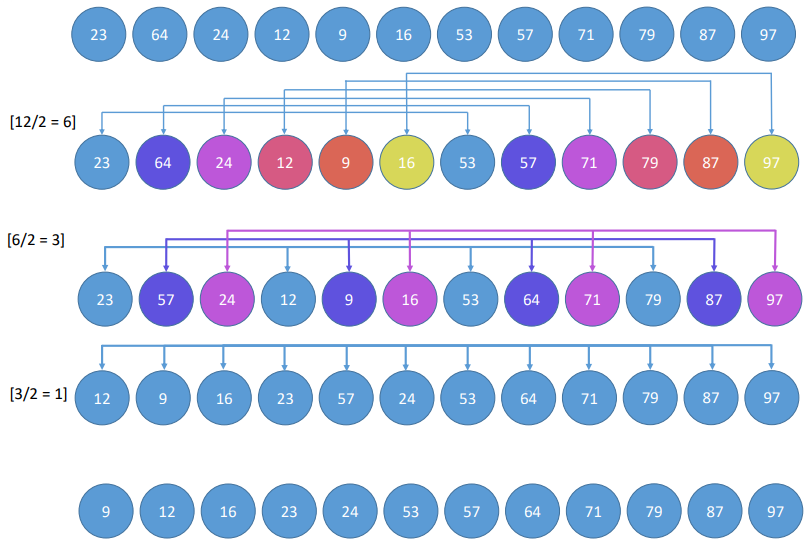• 該方法實質上是一種分組插入方法
• 希爾排序的時間性能優于直接插入排序，原因如下：
• 當數組初始狀態基本有序時，直接插入排序所需的比較和移動次數均較少
• 當n值較小時，n和n2的差別也較小，即直接插入排序的最好時間復雜度O(n)和最壞時間復雜度O()差別不大
• 在希爾排序開始時，增量較大，分組較多，每組的記錄數目少，故各組內直接插入較快，后來增量d逐漸縮小，分組數逐漸減少，而各組的記錄數目逐漸增多。但由于已經按d-1作為距離排過序，數組較接近于有序狀態，所以新的一趟排序過程也較快
• 希爾排序是不穩定的：由于分組的存在，相等的元素可能會分在不同組，導致它們的次序可能發生變化，因此希爾排序是不穩定的

### 實現代碼

/**
* @description: 希爾排序, 排序規則(從小到大)
* @param :
arr: 排序的數組
arrSize: 數組的大小
* @return:
* @author: Dongshao
*/
template<typename T>
void shellSort(T arr[], int arrSize)
{
// 初始化步長, 為數組的一半
int step = arrSize / 2;

int i, j;
// 只要步長大于0, 就進行一次希爾排序(內部其實是插入排序)
while(step > 0)
{
// 實際上根據step, 進行插入排序
for(i = step; i < arrSize; i += 1)
{
T temp = arr[i];

for(j = i - step; j >=0 && arr[j] > arr[i]; j -= step)
arr[j + step] = arr[j];

arr[j + step] = temp;
}

step /=2 ;
}
}

### 測試代碼如下

int main()
{
int arr[] = {5, 4, 1, 2, 7, 4};
shellSort<int>(arr, sizeof(arr) / sizeof(int));
std::cout << "arr: ";
for (const auto& elem : arr)
std::cout << elem << " ";
std::cout << std::endl;

return 0;
}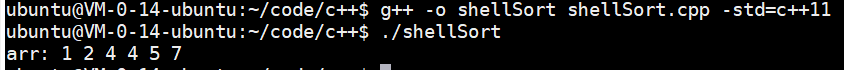# 五、歸并排序

### 原理與圖示

• 步驟一般為：
• 1.將序列中待排序數字分為若干組，每個數組分為一組
• 2.將若干組兩兩合并，保證合并后的組是有序的
• 3.重復第二步操作直到只剩下一組，排序完成
• 下面是一組圖解：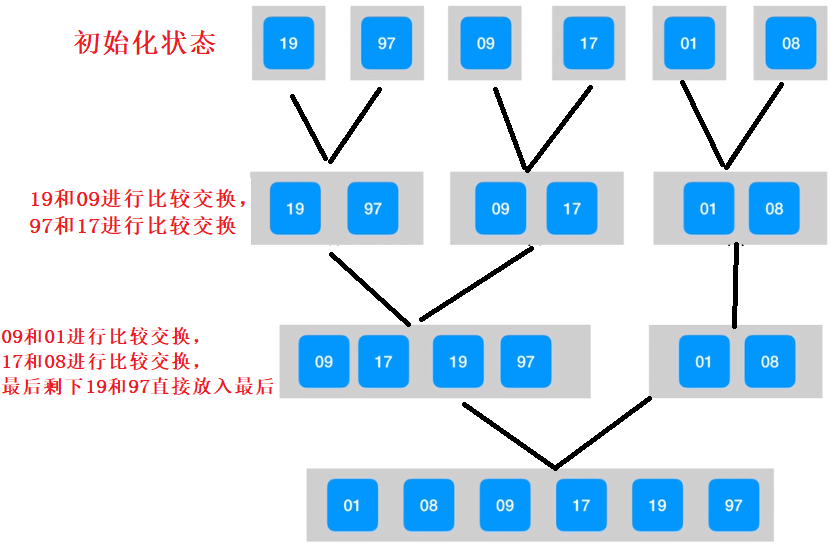### 實現代碼

template<typename T>
void _merge_sort(T *a, T *temp, int start, int middle, int end) {

int i = start, j = middle + 1, k = start;

while (i <= middle && j <= end) {
if (a[i] > a[j])
temp[k++] = a[j++];
else
temp[k++] = a[i++];
}

while (i <= middle)
temp[k++] = a[i++];
while (j <= end)
temp[k++] = a[j++];

for (i = start; i <= end; i++)
a[i] = temp[i];
}

template<typename T>
void merge_sort(T *a, T *temp, int start, int end) {
int middle;
if (start < end) {
middle = start + (end - start) / 2;

merge_sort(a, temp, start, middle);
merge_sort(a, temp, middle + 1, end);

_merge_sort(a, temp, start, middle, end);
}
}

### 測試代碼如下

int main()
{
int a[] = { 6,5,8,4,3,1 };
int temp = { 0 };
merge_sort(a, temp, 0, 5);
for (const auto& elem : temp)
std::cout << elem << " ";
}

?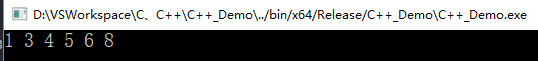# 六、快速排序

### 原理與圖示

• 快速排序是C.R.A.Hoare于1962年提出的一種劃分交換排序。它采用了一種分治的策略，通常稱其為分治法
• 基本思想：通過一趟排序將要排序的數據分割為獨立的兩部分，其中一部分的所有數據都比另外一部分的所有數據都要小，然后再按此方法對這兩部分數據分別進行快速排序，整個排序過程可以遞歸進行，以此達到整個數據變成有序序列

### 實現代碼1

• 下面的代碼中，在每次對序列進行排序時，我們選取序列最左側的元素作為排序的基本參照點
template<typename T>
void _quickSort(T arr[], int leftEnd, int rightEnd);

/**
* @description: 快速排序, 排序規則(從小到大)
* @param :
arr: 排序的數組
arrSize: 數組的大小
* @return:
* @author: Dongshao
*/
template<typename T>
void quickSort(T arr[], int arrSize)
{
_quickSort(arr, 0, arrSize - 1);
}

template<typename T>
void _quickSort(T arr[], int leftEnd, int rightEnd)
{
if (leftEnd >= rightEnd)
return;

int leftCursor = leftEnd;   // 記錄該區間最左索引
int rightCursor = rightEnd; // 記錄該區間最右索引
T pivot = arr[leftEnd];   // 我們以區間最左邊的值為基準(哨兵)

// 遍歷整個區間
while (leftCursor < rightCursor)
{
// 從右向左找出一個比哨兵小的值, 其索引為rightCurosr
while (leftCursor < rightCursor && arr[rightCursor] >= pivot)
rightCursor--;
// 然后將這個比哨兵小的值放到區間左邊, 之后將leftCursor++
// 備注, 此處不需要擔心arr[leftCursor]的值是否找不到了, 因為我們已經用pivot將其記錄下來了
if(leftCursor < rightCursor)
arr[leftCursor++] = arr[rightCursor];

// 從左向右找出一個比哨兵大的值, 其索引為leftCursor
while (leftCursor < rightCursor && arr[leftCursor] <= pivot)
leftCursor++;
// 然后比這個比哨兵大的值放到右邊, 之后將rightCursor
if(leftCursor < rightCursor)
arr[rightCursor--] = arr[leftCursor];

// 這一輪比較完了, 如果中間還有元素沒有進行比較, 進行下一輪
}

// 把哨兵的值放到leftCursor所指的位置(中間), 因為此時leftCursor是
arr[leftCursor] = pivot;

_quickSort(arr, leftEnd, rightCursor - 1);  // 對左區間遞歸排序
_quickSort(arr, rightCursor + 1, rightEnd); // 對右區間遞歸排序
}
• 測試代碼如下：
int main()
{
int arr[] = {5, 4, 1, 2, 7, 4};
quickSort<int>(arr, sizeof(arr) / sizeof(int));

std::cout << "arr: ";
for (const auto& elem : arr)
std::cout << elem << " ";
std::cout << std::endl;

return 0;
}

?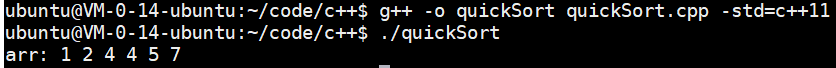### 實現代碼2

• 下面的代碼中是取自于《劍指Offer》中的實現，每次排序的時候，我們隨機從區間中取一個下標作為排序的參照點
#include <stdlib.h>
#include <stdexcept>

using namespace std;

// 生成一個隨機數
int RandomInRange(int min, int max)
{
int random = rand() % (max - min + 1) + min;
return random;
}

// 交換兩個值
int Swap(int *num1, int *num2)
{
int temp = *num1;
*num1 = *num2;
*num2 = temp;
}

// 最核心函數, 將start到end區間的元素切割為2部分, 并返回一個索引small,
// 其中在data[small]前面的元素都小于data[small], 在data[small]后面的元素都大于data[small]
int Partition(int data[], int length, int start, int end)
{
if(data == nullptr || length <= 0 || start < 0 || end >= length)
throw std::runtime_error("Invalid Parameters.");

// 隨機生成一個索引作為哨兵
int index = RandomInRange(start, end);

// 把data[index]這個元素放到最后, 用來作為比較標準
Swap(&data[index], &data[end]);

// 用來標識已經找到比data[end]小的索引, arr[small]及之前的所有元素都比data[end]小
int small = start - 1;

// 遍歷所有的數組
for(index = start; index < end; ++index)
{
// 如果遇到一個比data[index]小的
if(data[index] < data[end])
{
++small;
if(small != index)
Swap(&data[index], &data[small]);
}
}

// 將small向前移動1位
++small;
// 然后跟data[end]交換
Swap(&data[small], &data[end]);

return small;
}

void quickSort(int data[], int length, int start, int end)
{
if(start == end)
return;

int index = Partition(data, length, start, end);
if(index > start)
Partition(data, length, start, index - 1);
if(index < end)
Partition(data, length, index + 1, end);
}
• 測試代碼如下：
#include <iostream>

using namespace std;

int main()
{
int arr[] = {5, 4, 1, 2, 7, 4};
quickSort(arr, sizeof(arr) / sizeof(int), 0, sizeof(arr) / sizeof(int) - 1);

std::cout << "arr: ";
for (const auto& elem : arr)
std::cout << elem << " ";
std::cout << std::endl;

return 0;
}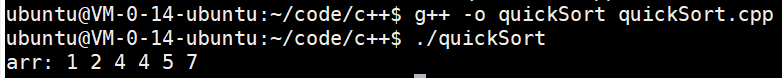05-076500
09-0414還能輸入1000個字符
01-15402
07-27736
08-31247
05-191030
05-08409
02-04104
12-16133
08-09160
??2020 CSDN 皮膚主題: 黑客帝國 設計師:上身試試
多乐彩